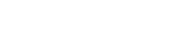One core part of the indypacs is an easy to extend modular interface to image analysis algorithms. Below is a set of examples and template files, that should help you putting together a module for your algorithm (e.g. in MATLAB)
.
Every module consists at least of two files.

1 - The ".imi" file It defines the input/output variables and assigns database fields to them. For example, if you want to perform an analysis of an x-ray image, you select a region of interest the coordinates of which need to be handed to MATLAB for the analysis and stored in the database for future reference. As different variables are necessary for different calculations, the imi file automatically generates the right database structure for each calculation.

Example: EvalHands_Script_v1.imi

EvalHands_Script_v1;matlab.exe -r MyMatlabScript –nosplash –nodesktop;1; Transferindypacs2Matlab.xml; TransferMatlab2indypacs.xml;
DBfield1; DBfield2; DBfield3;
DBfield4; DBfield5; DBfield6;

This imi file defines:

1. EvalHands_Script_v1 .... the name of the module
2. matlab.exe -r MyMatlabScript -nosplash -nodesktop;1; .... it starts matlab
3. MyMatlabScript .... this is the script it executes in MATLAB.
4. Transferindypacs2Matlab.xml .... that is the name of the xml data exchange file it will use to communicate the database values to MATLAB. Note that the xml will be generated by indypacs, so that is just the name that will be used.
5. TransferMatlab2indypacs.xml .... that is the name of the xml data exchange file it expects to get back from MATLAB.
6. DBfield 1-3 .... these are data base fields indypacs will send to MATLAB, in addition it always sends the dicom filename for a data base entry
7. DBfield 4-5 .... these are the data base fields indypacs expects to get back from MATLAB

Here  goes from indypacs to MATLAB

2 - The MATLAB code that actually performs the analysis. It takes the input values from the xml file, processes them automatically (you can take a coffee break or do other tasks in indypacs in the meantime) and eventually sends the calculated results back to indypacs, where you can easily work with them.

Example: MyMatlabScript.m

% For each dicom file perform some calculation
for i = 1:length(DicomsToAnalyse)
filename = DicomsToAnalyse(i).filename;
DBfield1 =DicomsToAnalyse(i).DBfield1;
DBfield2 = DicomsToAnalyse(i).DBfield2;
DBfield3 = DicomsToAnalyse(i).DBfield3;

% here your analysis takes place

DicomsToAnalyse(i).DBfield4 = 4;
DicomsToAnalyse(i).DBfield5 = 5;
DicomsToAnalyse(i).DBfield6 = 6;
end

xml_save('TransferMATLAB2indypacs',DicomsToAnalyse);

analysis module templates